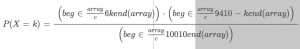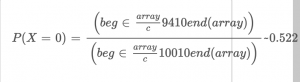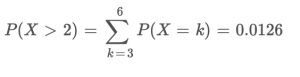## If X is the number of defective items in a randomly drawn sample of 10 items from the batch, find (a) P(X=0) and (b) P(X>2)?

Statistics and ProbabilityIf X is the number of defective items in a randomly drawn sample of 10 items from the batch, find (a) P(X=0) and (b) P(X>2)?2 years ago
Suppose that a batch of 100 items contains 6 that are defective and 94 that are not defective. If X is the number of defective items in a randomly drawn sample of 10 items from the batch, find (a) P(X=0) and (b) P(X>2)?
Observe that X in (0,1,2,3,4,5,6 ). Take any k in (0,1,2,3,4,5,6 ). The event X=k means that in our sample, we have taken k defective items and 10 - k non-detective items. HenceNow, we have thatandResult: Consider Hypergeometric distribution.